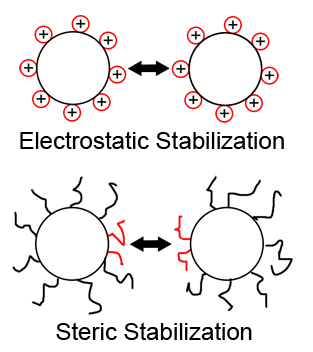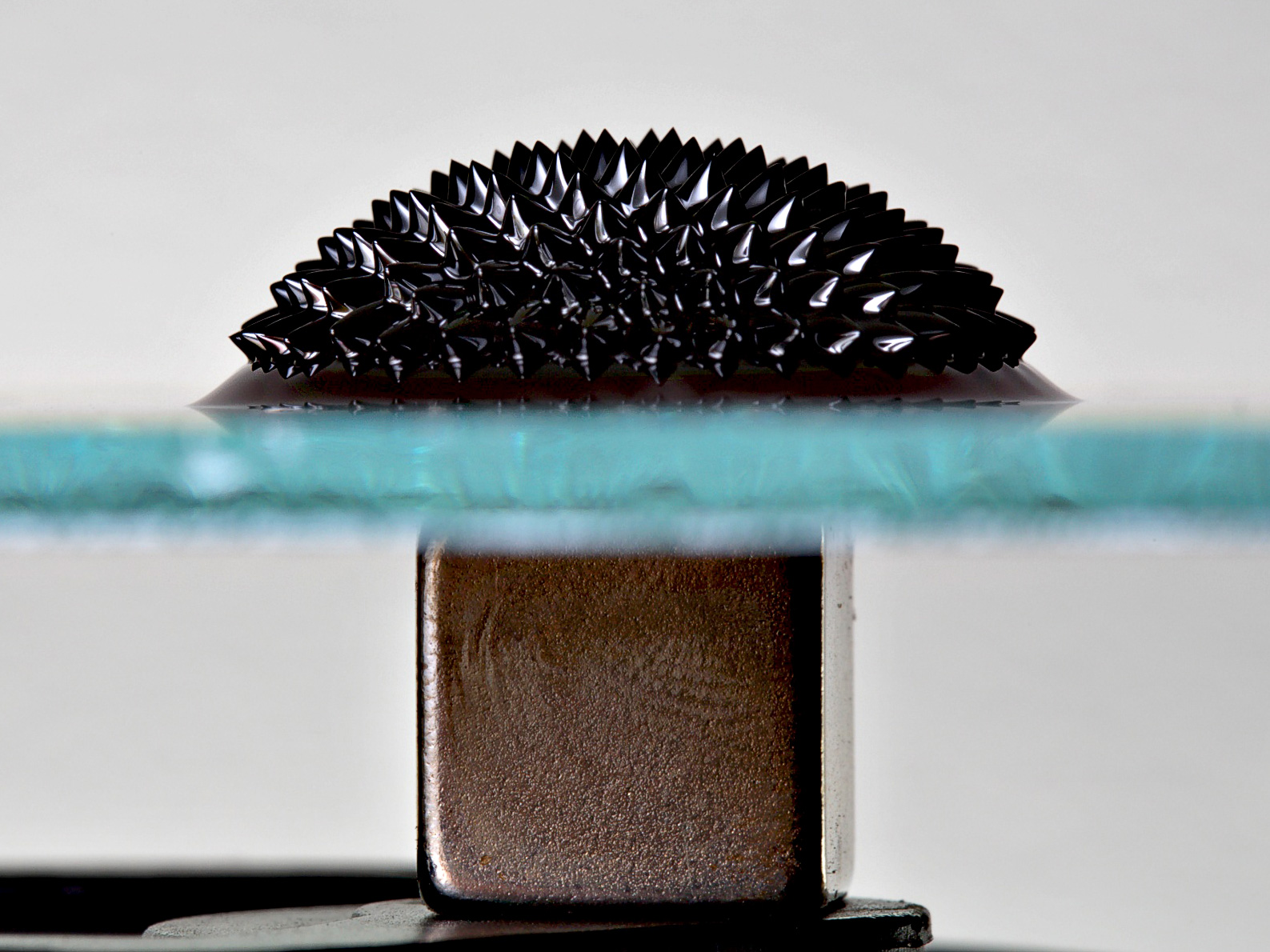# Ferrofluid - Part I¶

## Introduction¶

Ferrofluids are colloidal suspensions of ferromagnetic single-domain particles in a liquid carrier. As the single particles contain only one magnetic domain, they can be seen as small permanent magnets. To prevent agglomeration of the particles, due to van-der-Waals or magnetic attraction, they are usually sterically or electrostatically stabilized (see fig. 1). The former is achieved by adsorption of long chain molecules onto the particle surface, the latter by adsorption of charged coating particles. The size of the ferromagnetic particles are in the region of 10 nm. With the surfactant layer added they can reach a size of a few hundred nanometers. Have in mind that if we refer to the particle diameter $\sigma$ we mean the diameter of the magnetic core plus two times the thickness of the surfactant layer.

Some of the liquid properties, like the viscosity, the phase behavior or the optical birefringence can be altered via an external magnetic field or simply the fluid can be guided by such an field. Thus ferrofluids possess a wide range of biomedical applications like magnetic drug targeting or magnetic thermoablation and technical applications like fine positioning systems or adaptive bearings and dampers. In figure 2 the picture of a ferrofluid exposed to the magnetic field of a permanent magnet is shown. The famous energy minimizing thorn-like surface is clearly visible.Figure 1: Schematic representation of electrostatically stabilization (picture top) and steric stabilization (picture bottom)Figure 2: Real Ferrofluid exposed to an external magnetic field (neodymium magnet) 

## The Model¶

For simplicity in this tutorial we simulate spherical particles in a monodisperse ferrofluid system which means all particles have the same diameter $\sigma$ and dipole moment $\mu$. The point dipole moment is placed at the center of the particles and is constant both in magnitude and direction (in the coordinate system of the particle). This can be justified as the Néel relaxation times are usually negligible for the usual sizes of ferrofluid particles. Thus the magnetic interaction potential between two single particles is the dipole-dipole interaction potential which reads

\begin{equation*} u_{\text{DD}}(\vec{r}_{ij}, \vec{\mu}_i, \vec{\mu}_j) = \gamma \left(\frac{\vec{\mu}_i \cdot \vec{\mu}_j}{r_{ij}^3} - 3\frac{(\vec{\mu}_i \cdot \vec{r}_{ij}) \cdot (\vec{\mu}_j \cdot \vec{r}_{ij})}{r_{ij}^5}\right) \end{equation*}

with $\gamma = \frac{\mu_0}{4 \pi}$ and $\mu_0$ the vacuum permeability.

For the steric interaction in this tutorial we use the purely repulsive Weeks-Chandler-Andersen (WCA) potential which is a Lennard-Jones potential with cut-off radius $r_{cut}$ at the minimum of the potential $r_{cut} = r_{min} = 2^{\frac{1}{6}}\cdot \sigma$ and shifted by $\epsilon_{ij}$ such that the potential is continuous at the cut-off radius. Thus the potential has the shape

\begin{equation*} u_{\text{sr}}^{\text{WCA}}(r_{ij}) = \left\{ \begin{array}{ll} 4\varepsilon_{\text{lj}}\left[ \left( \frac{\sigma}{r_{ij}} \right)^{12} - \left( \frac{\sigma}{r_{ij}} \right)^6 \right] + \varepsilon_{\text{lj}} & r_{ij} < r_{cut} \\ 0 & r_{ij} \geq r_{cut} \\ \end{array} \right. \end{equation*}

where $r_{ij}$ are the distances between two particles. The purely repulsive character of the potential can be justified by the fact that the particles in real ferrofluids are sterically or electrostatically stabilized against agglomeration.

\begin{equation*} u(\vec{r}_{ij}, \vec{\mu}_i, \vec{\mu}_j) = u_{\text{sr}}(\vec{r}_{ij}) + u_{\text{DD}}(\vec{r}_{ij}, \vec{\mu}_i, \vec{\mu}_j) \end{equation*}

The liquid carrier of the system is simulated through a Langevin thermostat.

For ferrofluid systems there are three important parameters. The first is the volume fraction in three dimensions or the area fraction in two dimensions or quasi two dimensions. The second is the dipolar interaction parameter $\lambda$

\begin{equation} \lambda = \frac{\tilde{u}_{DD}}{u_T} = \gamma \frac{\mu^2}{k_BT\sigma^3} \end{equation}

where $u_{T} = k_BT$ is the thermal energy and $\tilde{u}_{DD}$ is the absolute value of the dipole-dipole interaction energy at close contact (cc) and head-to-tail configuration (htt) (see fig. 4) per particle, i.e. in formulas it reads

\begin{equation} \tilde{u}_{DD} = \frac{ \left| u_{DD}^{\text{htt, cc}} \right| }{2} \end{equation}

The third parameter takes a possible external magnetic field into account and is called Langevin parameter $\alpha$. It is the ratio between the energy of a dipole moment in the external magnetic field $B$ and the thermal energy

\begin{equation} \alpha = \frac{\mu_0 \mu}{k_B T}B \end{equation}

## Structure of this tutorial¶

The aim of this tutorial is to introduce the basic features of ESPResSo for ferrofluids or dipolar fluids in general. In part I and part II we will do this for a monolayer-ferrofluid, in part III for a three dimensional system. In part I we will examine the clusters which are present in all interesting ferrofluid systems. In part II we will examine the influence of the dipole-dipole-interaction on the magnetization curve of a ferrofluid. In part III we calculate estimators for the initial susceptibility using fluctuation formulas and sample the magnetization curve.

We assume the reader is familiar with the basic concepts of Python and MD simulations.

Remark: The equilibration and sampling times used in this tutorial would be not sufficient for scientific purposes, but they are long enough to get at least a qualitative insight of the behaviour of ferrofluids. They have been shortened so we achieve reasonable computation times for the purpose of a tutorial.

## Compiling ESPResSo for this Tutorial¶

For this tutorial the following features of ESPResSo are needed

#define EXTERNAL_FORCES
#define ROTATION
#define DIPOLES
#define LENNARD_JONES


Please uncomment them in the myconfig.hpp and compile ESPResSo using this myconfig.hpp.

## A Monolayer-Ferrofluid System in ESPResSo¶

For interesting ferrofluid systems, where the fraction of ferromagnetic particles in the liquid carrier and their dipole moment are not vanishingly small, the ferromagnetic particles form clusters of different shapes and sizes. If the fraction and/or dipole moments are big enough the clusters can interconnect with each other and form a whole space occupying network. In this part we want to investigate the number of clusters as well as their shape and size in our simulated monolayer ferrofluid system. It should be noted that a monolayer is a quasi three dimensional system (q2D), i.e. two dimensional for the positions and three dimensional for the orientation of the dipole moments.

### Setup¶

We start with checking for the presence of ESPResSo features and importing all necessary packages.

Now we set up all simulation parameters.

Note that we declared a lj_cut. This will be used as the cut-off radius of the Lennard-Jones potential to obtain a purely repulsive WCA potential.

Now we set up the system. The length of the simulation box is calculated using the desired area fraction and the area all particles occupy. Then we create the ESPResSo system and pass the simulation step. For the Verlet list skin parameter we use the built-in tuning algorithm of ESPResSo. After that we set up the thermostat which is, in our case, a Langevin thermostat to simulate in an NVT ensemble.

Hint: It should be noted that we seed the Langevin thermostat, thus the time evolution of the system is partly predefined. Partly because of the numeric accuracy and the automatic tuning algorithms of Dipolar P3M and DLC where the resulting parameters are slightly different every time. You can change the seed to get a guaranteed different time evolution.

Now we set up the interaction between the particles as a non-bonded interaction and use the Lennard-Jones potential as the interaction potential. Here we use the above mentioned cut-off radius to get a purely repulsive interaction.

Now we generate random positions and orientations of the particles and their dipole moments.

Hint: It should be noted that we seed the random number generator of numpy. Thus the initial configuration of our system is the same every time this script will be executed. You can change it to another one to simulate with a different initial configuration.

Now we add the particles with their positions and orientations to our system. Thereby we activate all degrees of freedom for the orientation of the dipole moments. As we want a two dimensional system we only allow the particles to translate in x- and y-direction and not in z-direction by using the fix argument.

Be aware that we do not commit the magnitude of the magnetic dipole moments to the particles. As in our case all particles have the same dipole moment it is possible to rewrite the dipole-dipole interaction potential to

\begin{equation} u_{\text{DD}}(\vec{r}_{ij}, \vec{\mu}_i, \vec{\mu}_j) = \gamma \mu^2 \left(\frac{\vec{\hat{\mu}}_i \cdot \vec{\hat{\mu}}_j}{r_{ij}^3} - 3\frac{(\vec{\hat{\mu}}_i \cdot \vec{r}_{ij}) \cdot (\vec{\hat{\mu}}_j \cdot \vec{r}_{ij})}{r_{ij}^5}\right) \end{equation}

where $\vec{\hat{\mu}}_i$ is the unit vector of the dipole moment $i$ and $\mu$ is the magnitude of the dipole moments. Thus we can only commit the orientation of the dipole moment to the particles and take the magnitude into account when calculating the dipole-dipole interaction with Dipolar P3M if we commit the magnitude to Dipolar P3M together with the prefactor $\gamma$ as a new prefactor

\begin{equation} \tilde{\gamma} = \gamma \mu^2 = \frac{\mu_0}{4\pi}\mu^2 = \lambda \sigma^3 k_BT \end{equation}

Of course it would also be possible to commit the whole dipole moment vectors to every particle and let the prefactor of Dipolar P3M unchanged ($\gamma$). In fact we have to do this if we want to simulate polydisperse systems.

Now we choose the steepest descent integrator to remove possible overlaps of the particles. Therefore we integrate until the energy of the whole system is lower than $5 k_BT$.

For the simulation of our system we choose the velocity Verlet integrator

To calculate the dipole-dipole interaction we use the Dipolar P3M method (see Ref. ) which is based on the Ewald summation. By default the boundary conditions of the system are set to conducting which means the dielectric constant is set to infinity for the surrounding medium. As we want to simulate a two dimensional system we additionally use the dipolar layer correction (DLC) (see Ref. ). As we add DipolarP3M to our system as an actor, a tuning function is started automatically which tries to find the optimal parameters for Dipolar P3M and prints them to the screen. The last line of the output is the value of the tuned skin.

Now we equilibrate the dipole-dipole interaction for some time

As we are interested in the cluster sizes and the number of clusters we now set up the cluster analysis with the distance criterion that a particle is added to a cluster if the nearest neighbors are closer than $1.3\cdot\sigma_{lj}$.

## Sampling¶

Now we sample our system for some time and do a cluster analysis in order to get an estimator of the cluster observables.

For the cluster analysis we create two empty lists. The first for the number of clusters and the second for their sizes.

We sample over 100 loops

As the system is two dimensional, we can simply do a scatter plot to get a visual representation of a system state. To get a better insight of how a ferrofluid system develops during time we will create a video of the development of our system during the sampling. If you only want to sample the system simply go to Sampling without animation

### Sampling with animation¶

To get an animation of the system development we have to create a function which will save the video and embed it in an html string.

Now we use the animation class of matplotlib to save snapshots of the system as frames of a video which is then displayed after the sampling is finished. Between two frames are 100 integration steps.

In the video chain-like and ring-like clusters should be visible which are possibly connected via Y- and X-links to each other. Some monomers should be also present.